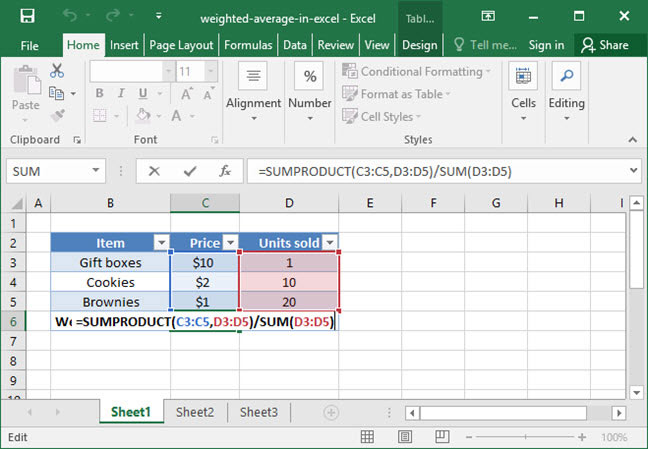# Finding a Weighted Average in Excel

Calculating a weighted average is possible in Excel using the SUMPRODUCT formula. Counterintuitively, the AVERAGE formula isn't involved at all! Let's take a look at what a weighted average is and how you calculate it in Excel.

## What is a weighted average?

A weighted average is an AVERAGE that takes into account not only the values of numbers, but also their weights. Consider the following example: Yesterday, SnackWorld sold 1 gift box at \$10.00, 10 cookies at \$2.00 each, and 20 brownies at \$1.00 each. It wants to calculate the weighted average price of each item that it sold.

If we wanted to calculate the simple, non-weighted AVERAGE price of products, we would do it like so:

`=AVERAGE(\$10.00, \$2.00, \$1.00)Output: \$4.33`

But the above formula doesn't really make sense: the average price per item sold isn't really \$4.33, because SnackWorld sold many more cookies than gift boxes. We need a formula that will take weighting (the number of items) sold into account rather than just value (the price of each item).

To solve this problem, we use a weighted average.

## The weighted average formula

The mathematical formula for taking a weighted average is as follows:

`((value_1 * weight_1) + (value_2 * weight_2)...)/(sum_of_weights)`

In plain English, to find the weighted average:

• Take the product of each value times its weight;
• Sum all of these products together; and
• Divide by the sum of the weightings.

Let's apply this formula to our SnackWorld example above to find the weighted average price per item sold. Remember, SnackWorld sold 1 gift box at \$10; 10 cookies at \$2 each; and 20 brownies at \$1 each. So our weighted average is:

`= ((\$10 * 1) + (\$2 * 10) + (\$1 * 20)) / (1 + 10 + 20)= (\$10 + \$20 + \$20) / (31)= \$50 / 31= \$1.61 / item`

That sounds more like it — our weighted average cost per item sold is \$1.61!

## Applying this to Excel

So how do we calculate a weighted average in Excel? If you're familiar with the SUMPRODUCT formula, you might have figured it out when we were talking about summing products together above: we can use SUMPRODUCT to sum a series of values and weightings, then divide by the SUM of weightings to get a weighted average!

Here's an example of the SUMPRODUCT formula in action, used to calculate a weighted average using the example we described above with SnackWorld's daily sales:`=SUMPRODUCT(C3:C5, D3:D5)/SUM(D3:D5)Step 1: =((C3*D3) + (C4*D4) + (C5*D5)) / SUM(D3:D5)Step 2: =(\$10 + \$20 + \$20) / 31Output: \$1.61`

Above, we first use the SUMPRODUCT formula to multiply each value by its weighting and then sum up the results. We then divide by the SUM of all the weightings to get the final weighted average price per unit sold.

This (SUMPRODUCT / SUM) formulation can be used to calculate weighted averages of any type, whether you want to find the average price per product weighted by units sold; the average student GPA weighted across different course types; or the average product review score rated across a number of different dimensions.

Have questions or comments on weighted averages? Sound off in the comments section below!

## Save an hour of work a day with these 5 advanced Excel tricks

Work smarter, not harder. Sign up for our 5-day mini-course to receive must-learn lessons on getting Excel to do your work for you.

• How to create beautiful table formatting instantly...
• Why to rethink the way you do VLOOKUPs...
• Plus, we'll reveal why you shouldn't use PivotTables and what to use instead...